# Excel IF statement with multiple conditions

The tutorial shows how to create multiple IF statements in Excel with AND as well as OR logic. Also, you will learn how to use IF together with other Excel functions.

In the first part of our Excel IF tutorial, we looked at how to construct a simple IF statement with one condition for text, numbers, dates, blanks and non-blanks. For powerful data analysis, however, you may often need to evaluate multiple conditions at a time. The below formula examples will show you the most effective ways to do this.

## How to use IF function with multiple conditions

In essence, there are two types of the IF formula with multiple criteria based on the AND / OR logic. Consequently, in the logical test of your IF formula, you should use one of these functions:

• AND function - returns TRUE if all the conditions are met; FALSE otherwise.
• OR function - returns TRUE if any single condition is met; FALSE otherwise.

To better illustrate the point, let's investigate some real-life formulas examples.

### Excel IF statement with multiple conditions (AND logic)

The generic formula of Excel IF with two or more conditions is this:

IF(AND(condition1, condition2, …), value_if_true, value_if_false)

Translated into a human language, the formula says: If condition 1 is true AND condition 2 is true, return value_if_true; else return value_if_false.

Suppose you have a table listing the scores of two tests in columns B and C. To pass the final exam, a student must have both scores greater than 50.

For the logical test, you use the following AND statement: AND(B2>50, C2>50)

If both conditions are true, the formula will return "Pass"; if any condition is false - "Fail".

`=IF(AND(B2>50, B2>50), "Pass", "Fail")`

Easy, isn't it? The screenshot below proves that our Excel IF /AND formula works right: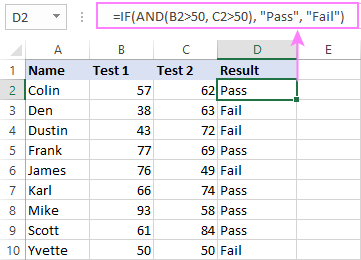In a similar manner, you can use the Excel IF function with multiple text conditions.

For instance, to output "Good" if both B2 and C2 are greater than 50, "Bad" otherwise, the formula is:

`=IF(AND(B2="pass", C2="pass"), "Good!", "Bad")`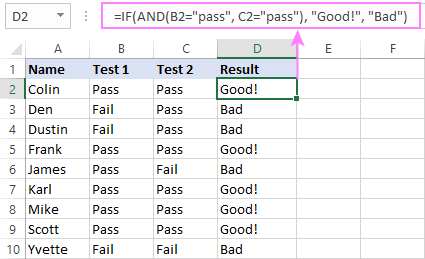Important note! The AND function checks all the conditions, even if the already tested one(s) evaluated to FALSE. Such behavior is a bit unusual since in most of programming languages, subsequent conditions are not tested if any of the previous tests has returned FALSE.

In practice, a seemingly correct IF statement may result in an error because of this specificity. For example, the below formula would return #DIV/0! ("divide by zero" error) if cell A2 is equal to 0:

`=IF(AND(A2<>0, (1/A2)>0.5),"Good", "Bad")`

The avoid this, you should use a nested IF function:

`=IF(A2<>0, IF((1/A2)>0.5, "Good", "Bad"), "Bad")`

### Excel IF function with multiple conditions (OR logic)

To do one thing if any condition is met, otherwise do something else, use this combination of the IF and OR functions:

IF(OR(condition1, condition2, …), value_if_true, value_if_false)

The difference from the IF / AND formula discussed above is that Excel returns TRUE if any of the specified conditions is true.

So, if in the previous formula, we use OR instead of AND:

`=IF(OR(B2>50, B2>50), "Pass", "Fail")`

Then anyone who has more than 50 points in either exam will get "Pass" in column D. With such conditions, our students have a better chance to pass the final exam (Yvette being particularly unlucky failing by just 1 point :)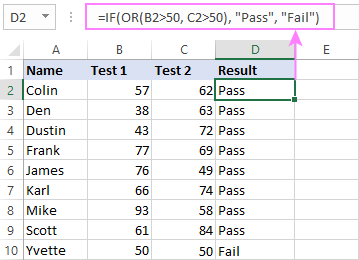Tip. In case you are creating a multiple IF statement with text and testing a value in one cell with the OR logic (i.e. a cell can be "this" or "that"), then you can build a more compact formula using an array constant.

For example, to mark a sale as "closed" if cell B2 is either "delivered" or "paid", the formula is:

`=IF(OR(B2={"delivered", "paid"}), "Closed", "")`

More formula examples can be found in Excel IF OR function.

### IF with multiple AND & OR statements

If your task requires evaluating several sets of multiple conditions, you will have to utilize both AND & OR functions at a time.

In our sample table, suppose you have the following criteria for checking the exam results:

• Condition 1: exam1>50 and exam2>50
• Condition 2: exam1>40 and exam2>60

If either of the conditions is met, the final exam is deemed passed.

At first sight, the formula seems a little tricky, but in fact it is not! You just express each of the above conditions as an AND statement and nest them in the OR function (since it's not necessary to meet both conditions, either will suffice):

`OR(AND(B2>50, C2>50), AND(B2>40, C2>60)`

Then, use the OR function for the logical test of IF and supply the desired value_if_true and value_if_false values. As the result, you get the following IF formula with multiple AND / OR conditions:

`=IF(OR(AND(B2>50, C2>50), AND(B2>40, C2>60), "Pass", "Fail")`

The screenshot below indicates that we've done the formula right: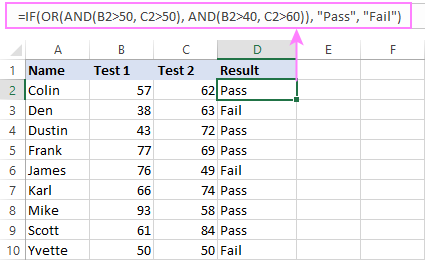Naturally, you are not limited to using only two AND/OR functions in your IF formulas. You can use as many of them as your business logic requires, provided that:

• In Excel 2007 and higher, you have no more than 255 arguments, and the total length of the IF formula does not exceed 8,192 characters.
• In Excel 2003 and lower, there are no more than 30 arguments, and the total length of your IF formula does not exceed 1,024 characters.

## Nested IF statement to check multiple logical tests

If you want to evaluate multiple logical tests within a single formula, then you can nest several functions one into another. Such functions are called nested IF functions. They prove particularly useful when you wish to return different values depending on the logical tests' results.

Here's a typical example: suppose you want to qualify the students' achievements as "Good", "Satisfactory" and "Poor" based on the following scores:

• Good: 60 or more (>=60)
• Satisfactory: between 40 and 60 (>40 and <60)
• Poor: 40 or less (<=40)

Before writing a formula, consider the order of functions you are going to nest. Excel will evaluate the logical tests in the order they appear in the formula. Once a condition evaluates to TRUE, the subsequent conditions are not tested, meaning the formula stops after the first TRUE result.

In our case, the functions are arranged from largest to smallest:

`=IF(B2>=60, "Good", IF(B2>40, "Satisfactory", "Poor"))`

Naturally, you can nest more functions if needed (up to 64 in modern versions).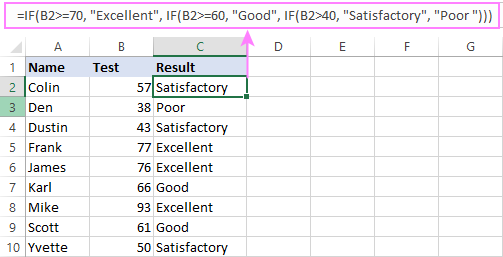## Excel IF array formula with multiple conditions

Another way to get an Excel IF to test multiple conditions is by using an array formula.

To evaluate conditions with the AND logic, use the asterisk:

IF(condition1) * (condition2) * …, value_if_true, value_if_false)

To test conditions with the OR logic, use the plus sign:

IF(condition1) + (condition2) + …, value_if_true, value_if_false)

To complete an array formula correctly, press the Ctrl + Shift + Enter keys together. In Excel 365 and Excel 2021, this also works as a regular formula due to support for dynamic arrays.

For example, to get "Pass" if both B2 and C2 are greater than 50, the formula is:

`=IF((B2>50) * (C2>50), "Pass", "Fail")`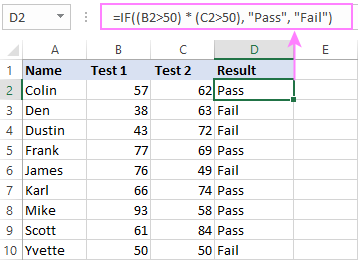In my Excel 365, a normal formula works just fine (as you can see in the screenshots above). In Excel 2019 and lower, remember to make it an array formula by using the Ctrl + Shift + Enter shortcut.

To evaluate multiple conditions with the OR logic, the formula is:

`=IF((B2>50) + (C2>50), "Pass", "Fail")`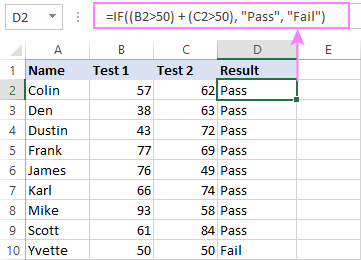## Using IF together with other functions

This section explains how to use IF in combination with other Excel functions and what benefits this gives to you.

### Example 1. If #N/A error in VLOOKUP

When VLOOKUP or other lookup function cannot find something, it returns a #N/A error. To make your tables look nicer, you can return zero, blank, or specific text if #N/A. For this, use this generic formula:

IF(ISNA(VLOOKUP(…)), value_if_na, VLOOKUP(…))

For example:

If #N/A return 0:

If the lookup value in E1 is not found, the formula returns zero.

`=IF(ISNA(VLOOKUP(E1, A2:B10, 2,FALSE )), 0, VLOOKUP(E1, A2:B10, 2, FALSE))`

If #N/A return blank:

If the lookup value is not found, the formula returns nothing (an empty string).

`=IF(ISNA(VLOOKUP(E1, A2:B10, 2,FALSE )), "", VLOOKUP(E1, A2:B10, 2, FALSE))`

If #N/A return certain text:

If the lookup value is not found, the formula returns specific text.

`=IF(ISNA(VLOOKUP(E1, A2:B10, 2,FALSE )), "Not found", VLOOKUP(E1, A2:B10, 2, FALSE))`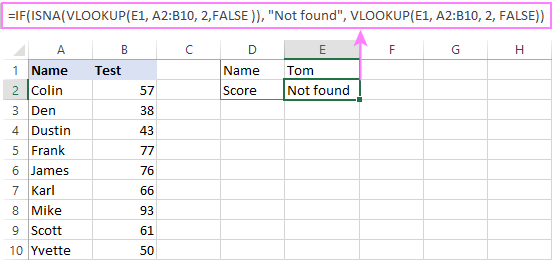For more formula examples, please see VLOOKUP with IF statement in Excel.

### Example 2. IF with SUM, AVERAGE, MIN and MAX functions

To sum cell values based on certain criteria, Excel provides the SUMIF and SUMIFS functions.

In some situations, your business logic may require including the SUM function in the logical test of IF. For example, to return different text labels depending on the sum of the values in B2 and C2, the formula is:

`=IF(SUM(B2:C2)>130, "Good", IF(SUM(B2:C2)>110, "Satisfactory", "Poor"))`

If the sum is greater than 130, the result is "good"; if greater than 110 – "satisfactory', if 110 or lower – "poor".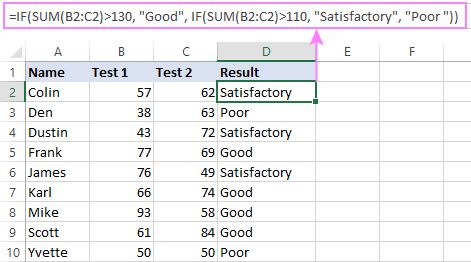In a similar fashion, you can embed the AVERAGE function in the logical test of IF and return different labels based on the average score:

`=IF(AVERAGE(B2:C2)>65, "Good", IF(AVERAGE(B2:C2)>55, "Satisfactory", "Poor"))`

Assuming the total score is in column D, you can identify the highest and lowest values with the help of the MAX and MIN functions:

`=IF(D2=MAX(\$D\$2:\$D\$10), "Best result", "")`

`=IF(D2=MAX(\$D\$2:\$D\$10), "Best result", "")`

To have both labels in one column, nest the above functions one into another:

`=IF(D2=MAX(\$D\$2:\$D\$10), "Best result", IF(D2=MIN(\$D\$2:\$D\$10), "Worst result", ""))`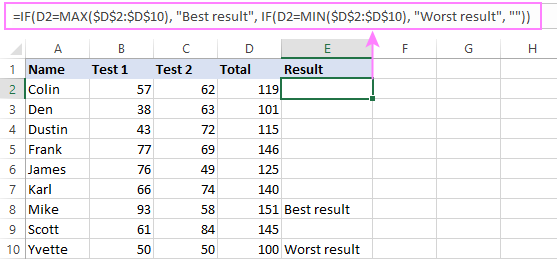Likewise, you can use IF together with your custom functions. For example, you can combine it with GetCellColor or GetCellFontColor to return different results based on a cell color.

In addition, Excel provides a number of functions to calculate data based on conditions. For detailed formula examples, please check out the following tutorials:

• COUNTIF - count cells that meet a condition
• COUNTIFS - count cells with multiple criteria
• SUMIF - conditionally sum cells
• SUMIFS - sum cells with multiple criteria

### Example 3. IF with ISNUMBER, ISTEXT and ISBLANK

To identify text, numbers and blank cells, Microsoft Excel provides special functions such as ISTEXT, ISNUMBER and ISBLANK. By placing them in the logical tests of three nested IF statements, you can identify all different data types in one go:

`=IF(ISTEXT(A2), "Text", IF(ISNUMBER(A2), "Number", IF(ISBLANK(A2), "Blank", "")))`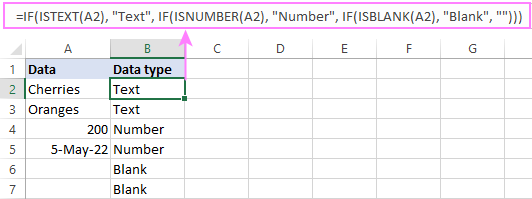### Example 4. IF and CONCATENATE

To output the result of IF and some text into one cell, use the CONCATENATE or CONCAT (in Excel 2016 - 365) and IF functions together. For example:

`=CONCATENATE("You performed ", IF(B1>100,"fantastic!", IF(B1>50, "well", "poor")))`

`=CONCAT("You performed ", IF(B1>100,"fantastic!", IF(B1>50, "well", "poor")))`

Looking at the screenshot below, you'll hardly need any explanation of what the formula does: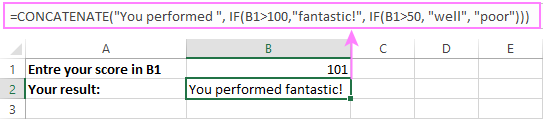## IF ISERROR / ISNA formula in Excel

The modern versions of Excel have special functions to trap errors and replace them with another calculation or predefined value - IFERROR (in Excel 2007 and later) and IFNA (in Excel 2013 and later). In earlier Excel versions, you can use the IF ISERROR and IF ISNA combinations instead.

The difference is that IFERROR and ISERROR handle all possible Excel errors, including #VALUE!, #N/A, #NAME?, #REF!, #NUM!, #DIV/0!, and #NULL!. While IFNA and ISNA specialize solely in #N/A errors.

For example, to replace the "divide by zero" error (#DIV/0!) with your custom text, you can use the following formula:

`=IF(ISERROR(A2/B2), "N/A", A2/B2)`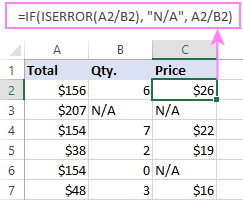And that's all I have to say about using the IF function in Excel. I thank you for reading and hope to see you on our blog next week!

Excel IF multiple criteria - examples (.xlsx file)

## You may also be interested in

1. So I have 2 columns with values that result in a final "grading" in a 3rd column.
The first column, G, has one of the following 9 values: 0,1,2,3,4A,4B,4C,5,6
The next Column, J, Has one of the following 3 values : "Benign/Negative", "Indeterminant/Atypical", "Malignant"
Dependent on the G/J combinations my 3 possible resultant values are : "Needs Review", "Yes - Pathology and Imaging are concordant", "No - Pathology and Imaging are discordant"

So when G=0 or 4B, or J="Indeterminant/Atypical", then "Needs Review"
When J="Benign/Negative" AND G>04 then "Yes - Pathology and Imaging are concordant"
If none of those conditions are met then "No - Pathology and Imaging are discordant"

How do I write this as IF statement?

• I apologize, part of the "AND" conditions did not make it in my question. The conditions are as follows:
G=0 or 4B, or J="Indeterminant/Atypical", then "Needs Review"
J="Benign/Negative" AND G>04 then "Yes - Pathology and Imaging are concordant"
If none of those conditions are met then "No - Pathology and Imaging are discordant"

• J="Benign/Negative", and G>04, "Yes - Pathology and Imaging are concordant"

• Hi! All the necessary information is in the article above. Also take a look at this guide: Excel Nested IF statements - examples, best practices and alternatives. For example:

=IF(OR(G1=0,G1="4B",J1="Indeterminant/Atypical"),"Needs Review", IF(AND(J1="Benign/Negative", G1>4),"Yes - Pathology and Imaging are concordant",""))

2. Hello,

I have three columns, column A for Status, column B for Reason, column C for Code.
I need to return a reason, and there are three different ones to Column B, depending on one of the status (there are two different ones) and one of the codes (there are several codes).

Thanks

3. Hi,
I'm not abe to get this one to work:
=IF(AND(K2>=0; K2=10; K2=20; K2<=40); "20-40"K2)))
Why?

• Look carefully at the conditions in the AND formula. They cannot be fulfilled at the same time.

• Hi Alexander,
The formula you see is not right, I'll try to pase again:

=IF(AND(K2>=0;K2=10;K2=20;K2<=40);"20-40"K2)))

• =IF(AND(K2>=0;K2=10;K2=20;K2<=40);"20-40"K2)))
I'm not able to send the complete formula

• Hi,
What I want is this:
6,98 0-10
12,56 10-20
29,58 20-40
Jan

If all 3 (A2.B2,C2) are green, only then overall status is green, if even 1 red then overall status is red, if all 3 amber, then overall status is red, for one or two ambers, overall status is amber

5. if Column C1 is above 0 give me Overtime or 0 give No OT, if column C1 is S give me Sick, If column C1 X give me Absent, if Column C1 is -1 give me Late Hours

• All the necessary information is in the article above.

6. How to add 2 cells, for example, if in a cell i have done drop down option with pass satisfied fail, another cell also same , for pass value 10, satisfied 7, fail zero) i need to add using if statement

• Sorry, I do not fully understand the task.
As it's currently written, it's hard to tell exactly what you're asking.

7. Need help to create a formula. Formula in L415, IF (J415<45,K415*2,0). I need to add a condition that if the result is less than a certain quantity, zero should be displayed

• Hi! If you have multiple conditions, try using nested IF statements. For example,

=IF(J415<45,IF((K415*2)>100,K415*2,0))

8. I need help with a formula please. I need it to be B10*4+D10+G10 but to also know that if F10=0 it should display the value of D10. Thank You

9. I have spreadsheets where several suppliers costs for products are in columns adjacent to each other. Each column has the supplier's name. There are many different products from lines 4 through to 100.I need the formula to choose the supplier with the lowest cost in new a column adjacent to these cost columns and to then also have a formula next to that lowest cost bringing in the name of that best cost supplier form the relevant column. My colleague uses the following for the best cost in for example column M. {=MIN(IF(G4:L40;G4:L4))} . When I copy this in a new spreadsheet it comes up as text and won't work and remains text. The formula for the best cost supplier he used =INDEX(\$G1:L1;MATCH)G4:L4;M4;0))
Can you please assist to give me generic formulae which I can use in every spreadsheet to assist in doing the calculations speedily.

• Hi! When you copy an array formula, don't copy the parentheses. Enter =MIN(IF(G4:L40;G4:L4)). It needs to be entered via Ctrl + Shift + Enter, not just Enter. You can read more about array formulas and their usage here.

10. Hi any help for this formula

=IF(K8>5000,(200), - working
=IF(K8<5000,K8*0.04)) - not working

• Hi! Try this formula:
=IF(K8<5000,K8*0.04)

11. I need to calculate the figure with 3 conditions as follows by using IF function:

IF % percentage achieved for a calculation between budget & actual figures is less than 100%, then figure is 0. however if achieved % is between 100%-170%, then take the achieved figure multiply by 10,000. Final condition, if % achieved is greater than 170%, then take 170% multiply by 10,000

I am unable to add the 2nd condition of between 100%-170%, in the same formula

12. Amazing exercises for practice, thank you so much!

13. Having difficulty with IF AND function need help

14. Dear Team,
Thank you for the great jobs.
I have rows with 3 column A 2 - B2 - C2
I want to highlight in green the rows with one cell or more with a value between 5 and 10.99 AND one cell or more with a value between 15 and 25.99
Thank you.
Best regards.

15. Can you have a formula that shows if B1 is empty then show "To Load" if there is a date then "loaded", but if D1 has a date then show "Shipped" and if H1 has a date then shows "Arrived"

• I also need to know the formula for two column condition

16. If the status of my data is INACTIVE, what formula can I use for it to automatically countdown 30 days?

17. what is the formula to determine if a given row has more than one column with a positive integer? the top row below (from a pivot table) has 7 columns, 64 is the total for the row. 2 of the 5 columns have data, so would want this to be a Yes.
the second row only has one entry, so would want this to be a No.
EX:
user name 17 47 64
user name 2 2

• Hi! If I understand your task correctly, the following formula should work for you:

=SUMPRODUCT(IFERROR(INT(A1:G1)=A1:G1,0)*(A1:G1>0))>1

18. i have a column A with100 rows each having a unique value eg (XXX YYY) , I have another column B with say 50 unique values YYY, now I want to find column B value row by row from the 100 rows in Column A and if there is match , copy the value from column A in column C, next to value in column B
I hope i am able to describe my requirement :-) Below one example, any help to get this would be highly appreciated thank you - vaibhav

A B C
XXX YYY YYY XXX YYY
XX1 YY2 YY2 XXX YY2
XX2 YY6 YY3 XX7 YY3
XX3 YY8 YY4 XX6 YY4
XX4 YY7 YY5
XX5 YY2 YY6 XX2 YY6
XX6 YY4 YY7 XX4 YY7
XX7 YY3 YY8 XX3 YY8

• Hi! To get the desired value, use the INDEX MATCH function. To find a partial match in a text string, use these instructions: How to find substring in Excel.
The formula might look like this:

=INDEX(A2:A10,MATCH(TRUE,ISNUMBER(SEARCH(B2,A2:A10)),0))

19. Hello, need your assistance on how can I formulate a shortcut (or formula) with an automatic answer in C?
All answers are 0 except for both NO which is 1.

A B C
YES NO 0
NO NO 1
YES YES 0
NO YES 0
YES YES
YES NO

Thank you so much in advance.

• Thank you so much! :)

20. need a formula in excel if any joined before 31/12/2022 are eligible for increment also any one joined up to 31/03/2023 but rejoinees are also eligbile for increment. Any one joined after 31/12/2022 upto 31/103/2023 but not rejoinees are not eligible for increment

21. If A2, B2 & C2 having Alphanumeric number (A0013) then result come (ok) and A2, B2 & C2 if any column missing alphanumeric (like 0 or NA) then result come Delete.